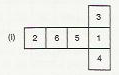# Dice are cubes where the numbers on the opposite faces must total 7.

Question:

Dice are cubes where the numbers on the opposite faces must total 7. Which of the following are dice?Solution:

Among the given figures, only figure (i) is a dice.

This is because if we fold the given net from the edges, we'll get a cube in which the sum of the opposite faces is 7 .